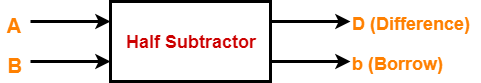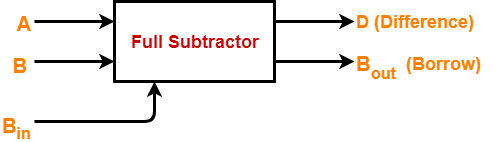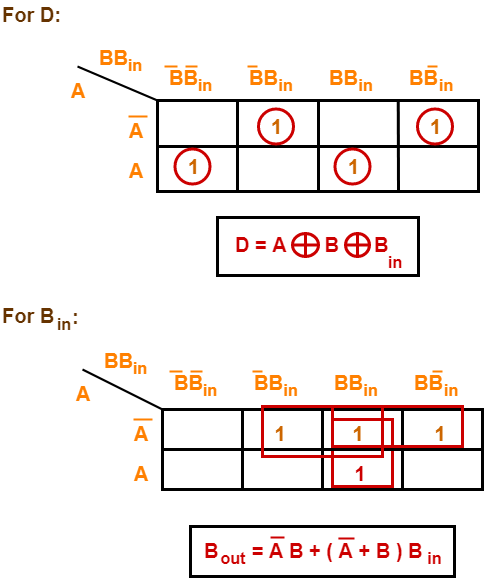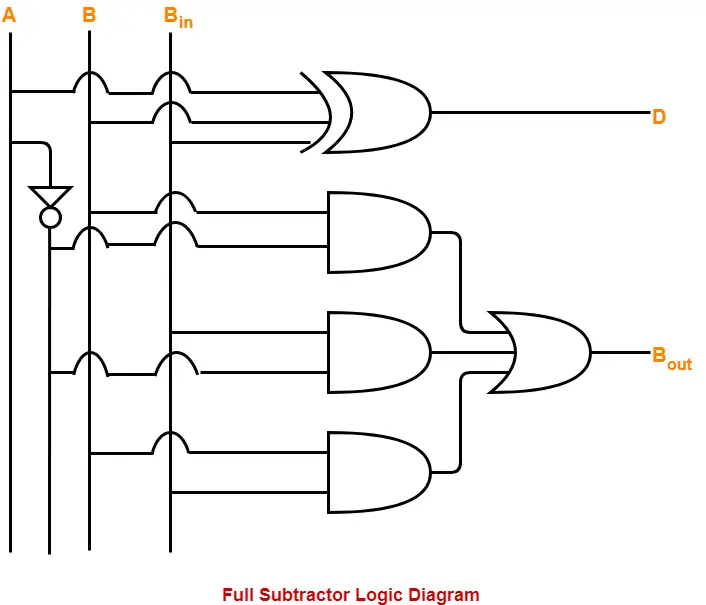# Full Subtractor | Definition | Circuit Diagram | Truth Table

## Half Subtractor-

Before you go through this article, make sure that you have gone through the previous article on Half Subtractor.

We have discussed-

• Half Subtractor is used for the purpose of subtracting two single bit numbers.
• Half subtractors have no scope of taking into account “Borrow-in” from the previous circuit.
• To overcome this drawback, full subtractor comes into play.## Full Subtractor-

• Full Subtractor is a combinational logic circuit.
• It is used for the purpose of subtracting two single bit numbers.
• It also takes into consideration borrow of the lower significant stage.
• Thus, full subtractor has the ability to perform the subtraction of three bits.
• Full subtractor contains 3 inputs and 2 outputs (Difference and Borrow) as shown-## Designing a Full Subtractor-

Full subtractor is designed in the following steps-

### Step-01:

Identify the input and output variables-

• Input variables = A, B, Bin (either 0 or 1)
• Output variables = D, Bout where D = Difference and Bout = Borrow

### Step-02:

Draw the truth table-

 Inputs Outputs A B Bin Bout (Borrow) D (Difference) 0 0 0 0 0 0 0 1 1 1 0 1 0 1 1 0 1 1 1 0 1 0 0 0 1 1 0 1 0 0 1 1 0 0 0 1 1 1 1 1

### Step-03:

Draw K-maps using the above truth table and determine the simplified Boolean expressions-### Step-04:

Draw the logic diagram.

The implementation of full adder using 1 XOR gate, 3 AND gates, 1 NOT gate and 1 OR gate is as shown below-To gain better understanding about Full Subtractor,

Watch this Video Lecture

Next Article- Ripple Carry Adder

Get more notes and other study material of Digital Design.

Watch video lectures by visiting our YouTube channel LearnVidFun.

Summary
Article Name
Full Subtractor | Definition | Circuit Diagram | Truth Table
Description
Full Subtractor is a combinational logic circuit used for the purpose of subtracting two single bit numbers with a borrow. Full Subtractor Definition, Block Diagram, Truth Table, Circuit Diagram, Logic Diagram, Boolean Expression and Equation are discussed. Full Subtractor overcomes the limitation of Half Subtractor.
Author
Publisher Name
Gate Vidyalay
Publisher Logo